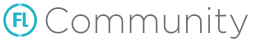# Away3d has an example of using dae to play animation

Hi, everyone, I recently researched away3d and chose Blender as a modeling tool, but I didn’t find a way to control the dea object to play animations in away3d.

Away3D library seems to have many problems, I will get an error when compiling:

``````openfl__\$Vector_Vector_\$Impl_\$.ofArray_String = function(array) {
var vector = new openfl__\$Vector_Vector_\$Impl_\$();
var ` = 0;
var `1 = array.length;
while` < `1 {
var i = `++;
vector.set(i,array[i]);
}
return vector;
};
openfl__\$Vector_Vector_\$Impl_\$.ofArray_Int = function(array) {
var vector = new openfl__\$Vector_Vector_\$Impl_\$();
var ` = 0;
var `1 = array.length;
while` < `1 {
var i = `++;
vector.set(i,array[i]);
}
return vector;
};
``````

This happens in HTML5, compiled with haxe.rc.5, and this happens if Vector.arrayOf is used.